## E1.10: Section 6 Part 2

Start exploring. How does changing h change the graph?

Start by changing h to -2.

That makes the spreadsheet look like the illustration below.

 A B C D E F G H 1 x y 2 -6 36 2 a 3 -5 22 -2 h 4 -4 12 4 k 5 -3 6 6 -2 4 7 -1 6 8 0 12 9 1 22 10 2 36 11 3 54 12 4 76 13 5 102 14 6 132 15 16

Now we can notice that, when $h=3$, the lowest point on the graph is at $x=3$, and when $h=-2$, then the lowest point on the graph is at $x=-2$.

This suggests that maybe the value that is subtracted from x in the original formula is the one that determines where the lowest y-value is – that is, where the lowest point on the graph is.

Try $h=0$, $h=4$, and $h=-3$.

 $h=0$ (leaving $a=2$ and $k=4$) $h=4$ (leaving $a=2$ and $k=4$) $h=-3$ (leaving $a=2$ and $k=4$)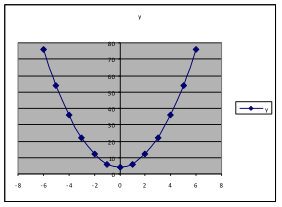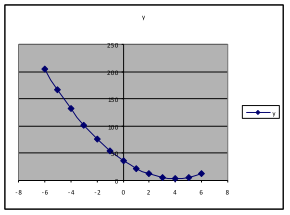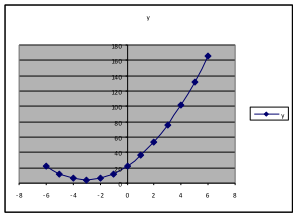Do these results support the conjecture we made in the previous sentence?   Answer: Yes.

Example 21.   Using the same formula and spreadsheet as in Example 18, use the values $a=1$, $h=0$, and explore the effect of changing k.

 $k=4$ (leaving $a=1$ and $h=0$) $k=0$ (leaving $a=1$ and $h=0$) $k=-7$ (leaving $a=1$ and $h=0$)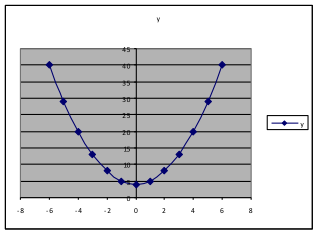We find that changing k alone changes how far up or down the lowest point on the graph is. It appears that the y-value of that lowest point is k.

Example 22.   Using the same formula and spreadsheet as in Example 17, use $h=0$ and $k=0$, and explore the effect of changing a.

 $a=1$ (with $h=0$ and $k=0$) $a=3$ (with$h=0$ and $k=0$) $a=-3$ (with $h=0$ and $k=0$)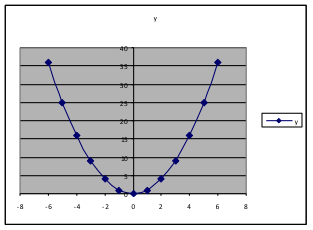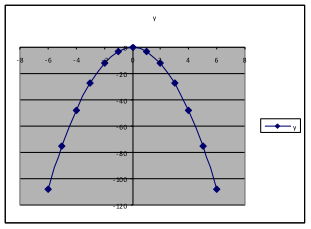We find that changing a from a positive to a negative number makes the graph change from opening upward to opening downward. Making a larger (from 1 to 3) changes how large the y-values are, so that the y-values for $a=3$ are three times as large as those when $a=1$.